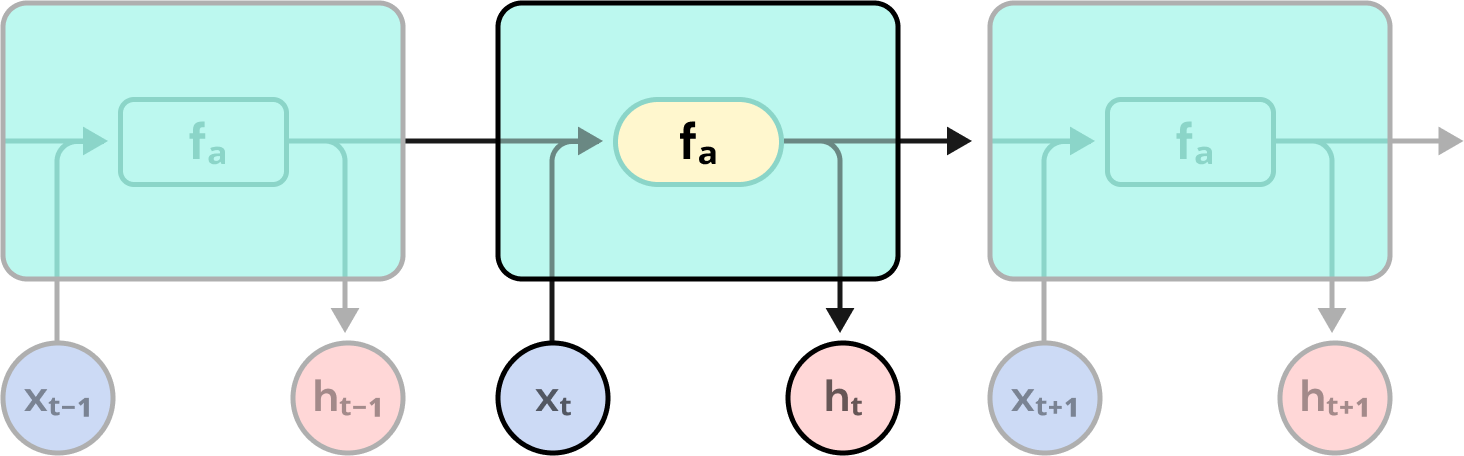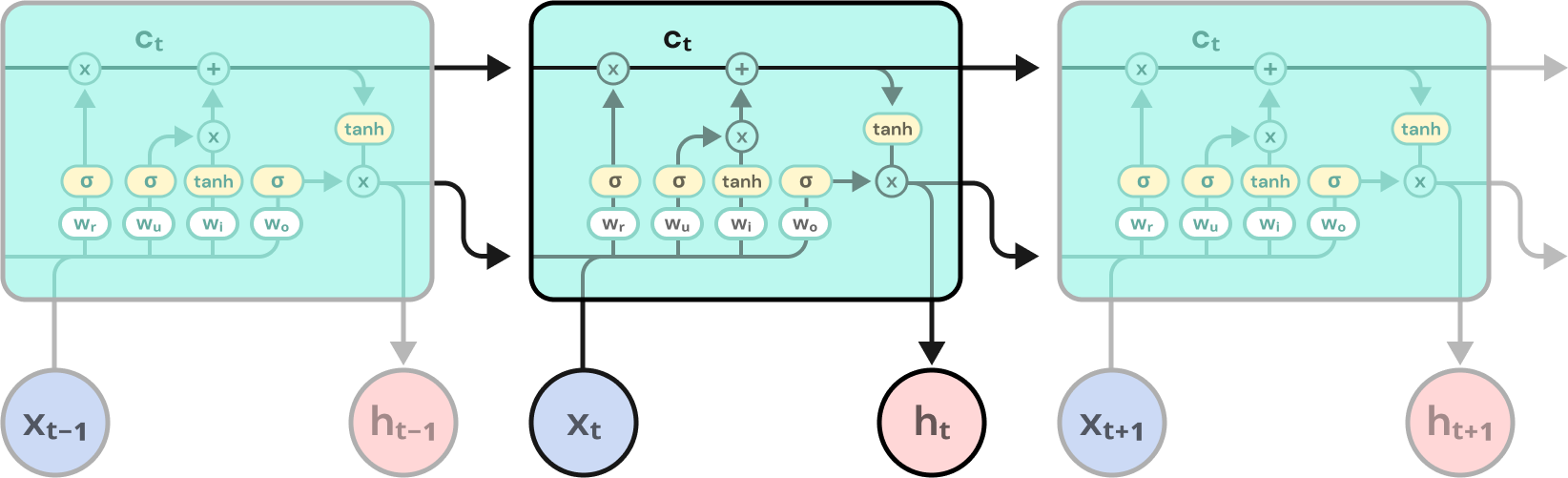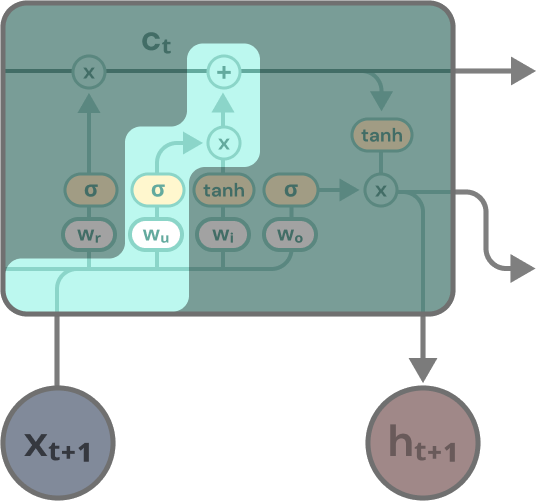# The Long Short-Term Memory Network

##### Technical Consultant

LSTMs are widely used in machine learning now. What are they? How do they work?

A Long Short-Term Memory (LSTM) neural network is a special kind of recurrent neural network that fixes the exploding and vanishing gradients problem. Since its proposal by Hochreiter and Schmidhuber in 1997, LSTMs have seen widespread adoption in the machine learning space. But how do they work?

### Recurrent Neural Networks

Before discussing LSTMs further, let’s review recurrent neural networks (RNN). A simple feedforward network consists of an input layer, a hidden layer, and an output layer. Given an input X, it gives you an output (i.e., predicts) Y. Each Y is only dependent on the X that produces it. In other words, every XY pair is independent, and only X is needed to predict Y. However, there are many cases where temporal dependencies exist. So, to accurately predict Y at time t (Yₜ), you need more than just X at time t (Xₜ), but also past X values (e.g., Xₜ₋₁, Xₜ₋₂, Xₜ₋₃). For example, as you read this post, your understanding of each word is informed by the preceding ones.

A feedforward network cannot account for temporal dependencies. By adding a feedback loop to the feedforward network, we give it a memory. This feedback loop recurs the network state of one run of the network to the next run. For example, Xₜ₋₁’s network state is fed back into the network and informs Xₜ. Simply put, the network “remembers” its previous outputs and can account for temporal relationships. That’s an RNN.

You can think of an RNN as a series of feedforward networks that feed into one another sequentially.

### Backpropagation Through Time

Like how feedforward networks training uses backpropagation, RNNs use backpropagation through time. Backpropagation through time is the application of backpropagation training to RNNs applied to sequential data.

To conceptualize the training process, let’s imagine a simple RNN with an input layer, one hidden layer, and an output layer. The network recurs the hidden layers output back onto itself. There are weights between the input and hidden layers (Wᵢₕ), the hidden and output layers (Wₕₒ), and the hidden layer and itself (Wₕₕ).

An RNN can be “unrolled”. In other words, you can think of an RNN as a series of feedforward networks that feed into one another sequentially. By removing the recursive connection from the simple RNN we described in the previous paragraph, we get a simple feedforward network we’ll call “FFN”. We wish to input a data series into the network (e.g., X₁ to Xₙ). We input X₁ into FFN₁ and weighted with Wᵢₕ. FFN₁’s hidden layer gets to work and outputs the hidden vector h₁. h₁ is then weighted with Wₕₒ to produce the output of the network (Y₁). A copy of h₁ is weighted with Wₕₕ and sent to the next feedforward network (FFN₂). FFN₂ is identical to FFN₁ except that it also takes in the weighted h₁. FFN₂’s hidden layer takes in the weighted h₁ and X₂ and produces h₂. The rest of the process is the same. Each FFNₜ feds into the next one (i.e., FFNₜ₊₁) until the entire data sequence or the selected number of “time steps” is processed (sequential inputs consumed). All FFNs share the weights (i.e., Wᵢₕ, Wₕₒ, and Wₕₕ).

Hochreiter and Schmidhuber’s LSTM solves both problems with gates.

### Long Short-Term Memory

Tricks like gradient clipping can fix exploding gradients, but vanishing gradients necessitate a change in the RNN architecture. Hochreiter and Schmidhuber’s LSTM solves both problems with gates. The LSTM is a special kind of recurrent neural network that uses a more complex “cell” structure. A cell is one processing unit of an LSTM or RNN (i.e., timestep). For example, an RNN that processes five timesteps (i.e., t - 4 to t) has five cells once unrolled.

A cell in a typical RNN is pretty simple. It takes in the hidden state from the previous time step (i.e., hₜ₋₁) and the current input (i.e., Xₜ), concatenates them, and multiplies them by their respective weights (i.e., Wₕₕ and Wᵢₕ). The activation function takes the weighted inputs and produces the current timestep’s hidden state (i.e., hₜ). hₜ feds into the next cell. A copy of hₜ is weighted with the output weight (i.e., Wₕₒ) and emitted as the cell’s output (i.e., Yₜ). Expressed as equations, these operations are:

hₜ = fₐ(hₜ₋₁, Xₜ)

Yₜ = Wₕₒhₜ

With fₐ being the activation function of the cell. If we assume that the activation function is tanh, then hₜ’s equation becomes:

hₜ = tanh(Wₕₕhₜ₋₁+WᵢₕXₜ)A three-timestep RNN depicted as a series of cells. The RNN cell is simple, containing only the activation function (fₐ).

An LSTM’s cell consists of four different gates (i.e., networks) interacting. The gates are: the forget gate, the update gate, the input gate, and the output gate. As alluded to earlier, each gate is a network in and of itself. Each gate has weights, an activation function, and two outputs. All of the gates share the weights. Each LSTM cell takes in three inputs: the previous timestep’s hidden state (i.e., hₜ₋₁), the previous timestep’s cell state (i.e., cₜ₋₁), and the current timestep’s input (i.e., Xₜ). The cell produces two outputs: the current timestep’s hidden state (i.e., hₜ), and the current timestep’s cell state (i.e., cₜ). hₜ₋₁ and Xₜ are roughly analogous to the hₜ₋₁ and Xₜ in RNNs. The cell state (i.e., cₜ) is the long-term memory component of the LSTM. The equations for the LSTM are:

i=tanh(Wᵢ(hₜ₋₁, Xₜ))

f=𝞼(Wᵣ(hₜ₋₁, Xₜ))

o=𝞼(Wₒ(hₜ₋₁, Xₜ))

u=𝞼(Wᵤ(hₜ₋₁, Xₜ))

cₜ=f⊗cₜ₋₁+iu

hₜ=o⊗tanh(cₜ)

i, f, o, and u are the outputs of the input, forget, output, and update gates, respectively. Wᵢ, Wᵣ, Wₒ, and Wᵤ are the weights for the input, forget, output, and update gates, respectively. 𝞼 and tanh are the activation functions for their respective equations. ⊗ is pointwise multiplication.The LSTM call is quite a bit more complicated than the RNN cell. It consists of four gates: the forget gate, the input gate, the update gate, and the output gate.

Let’s go through the operation of an LSTM and its gates to see how they function. The LSTM cell takes in cₜ₋₁, hₜ₋₁, and Xₜ. The cell concatenates the hₜ₋₁ and Xₜ vectors, and copies of the concatenated vector feeds into each gate. The forget gate takes in the concatenated hₜ₋₁ and Xₜ vector, weights it with Wᵣ, and pushes it through its activation function (i.e., 𝞼). The result is a vector of values between 0 and 1 that are pointwise multiplied with cₜ₋₁, zeroing out or attenuating various values in cₜ₋₁. Thus, the forget gate removes stuff from long-term memory or makes the LSTM “forget” parts of the long-term memory.

The input gate takes in the concatenated hₜ₋₁ and Xₜ vector, weights it with Wᵢ, and pushes it through its activation function (i.e., tanh), getting a vector of values between -1 and 1. So the input gate produces a “candidate memory” that might get written into cₜ.

The update gate takes in the concatenated hₜ₋₁ and Xₜ vector, weights it with Wᵤ, and pushes it through its activation function (i.e., 𝞼), getting a vector of values between 0 and 1. Afterward, that output and the input gate’s output (i.e., i) are pointwise multiplied, creating the actual memory to write into cₜ. Thus, the update gate creates a “memory mask” that determines which parts of the “candidate memory” to remember.The update gate creates a "memory mask" to apply to the input gate's "candidate memory" to determine the actual memory to remember.

Finally, the output gate takes in the concatenated hₜ₋₁ and Xₜ vector, weights it with Wₒ, and pushes it through its activation function (i.e., 𝞼), getting a vector of values between 0 and 1. The resulting vector is multiplied, element-wise, by cₜ pushed through a tanh. This result is the cell’s hidden state output (i.e., hₜ). As with RNNs, hₜ is passed onto the next cell and emitted as the current cell’s output. So the output gate is an attention mechanism that works by producing an “attention mask” to focus the cell on the relevant bits of the memory.

Like hₜ, cₜ feeds into the next cell, unweighted, as the new long-term memory. Unlike hₜ, cₜ is never emitted as output and stays confined within the cells.